## Friday, July 19, 2013

### 2Partition Index for MSSQL

Recently, I got confuse about the partition index, so I decided to do some testing about it.

## Create the sample database

`use master`
`CREATE DATABASE [PARTITION_DEMO] ON  PRIMARY `
`( NAME = N'PARTITION2011_DATA', FILENAME = N'C:\DATA\PARTITION2011_DATA.mdf' , SIZE = 4096KB , MAXSIZE = UNLIMITED, FILEGROWTH = 1024KB ),`
`FILEGROUP FG2012`
`(NAME = 'PARTITION2012_DATA',FILENAME ='C:\DATA\PARTITION2012_DATA.ndf',SIZE =4096KB,MAXSIZE=UNLIMITED,FILEGROWTH=1024KB )`
` LOG ON `
`( NAME = N'PARTITION_LOG', FILENAME = N'C:\DATA\PARTITION_LOG.ldf' , SIZE = 2048KB  , FILEGROWTH = 10%);`

## Create partition function and scheme

`CREATE PARTITION FUNCTION partiton_demo_f (datetime)`
`AS RANGE RIGHT FOR VALUES ('9/01/2011','10/01/2011', '11/01/2011', '12/01/2011',`
`               '1/01/2012', '2/01/2012', '3/01/2012', '4/01/2012',`
`               '5/01/2012', '6/01/2012', '7/01/2012', '8/01/2012');`
`GO`
` `
`CREATE PARTITION SCHEME partiton_demo_s`
`AS PARTITION partiton_demo_f`
`TO ([PRIMARY], [PRIMARY], [PRIMARY]`
`, [PRIMARY], [FG2012], [FG2012]`
`, [FG2012], [FG2012], [FG2012]`
`, [FG2012], [FG2012], [FG2012]`
`, [FG2012], [FG2012]);`
`GO`

## create the test table and insert some data

`Create Table dbo.orders`
`(`
`      order_id  int Identity(1,1)   Not Null`
`    ,  product_name char(1000) Not Null `
`    , orderDate datetime            Not Null`
`    , orderData smalldatetime       Not Null`
` `
`) On partiton_demo_s(orderDate);`
`Go`
` `
` `
`Set NoCount On;`
` `
`Declare @endDate datetime = '2011-08-01';`
`Declare @randomString varchar(255);`
` `
`While @endDate < '2012-09-01'`
`Begin`
`    SELECT @randomString = CONVERT(varchar(255), NEWID());`
`    Insert Into dbo.orders `
`    Select @randomString,@endDate, @endDate`
` `
`    Set @endDate = DATEADD(minute, 3, @endDate);`
` `
`End;`

## Check the data distribution

`use PARTITION_DEMO:`
` `
`SELECT * FROM sys.partitions WHERE OBJECT_ID = OBJECT_ID('dbo.orders');`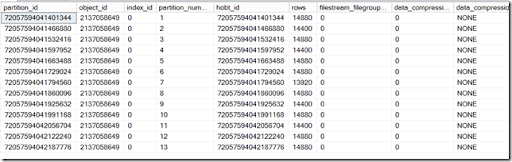`Select i.name`
`    , i.index_id`
`    , p.partition_number`
`    , p.rows`
`From sys.partitions As p`
`Join sys.indexes As i`
`    On p.object_id = i.object_id `
`   And p.index_id = i.index_id`
`Where p.object_id = object_id('orders')`
`Order By i.index_id, p.partition_number;`

Because there are no indexes, so the index_id is 0 , meaning it is heap.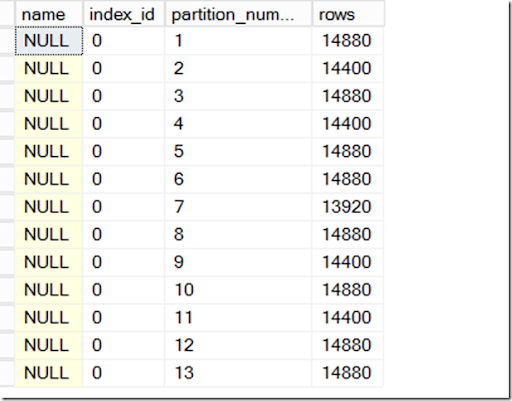## Create the partition index

`CREATE NONCLUSTERED INDEX ix_orders_partitioned`
`    ON dbo.orders(order_id)`
`     ON partiton_demo_s(orderDate);`

Now check the index size again

`SELECT i.name`
`    , i.index_id`
`   ,p.rows`
`   ,p.partition_id`
`   ,a.used_pages*8 AS 'Used space(KB)'`
`FROM sys.partitions AS p`
`JOIN sys.indexes AS i`
`    ON p.object_id = i.object_id `
`   AND p.index_id = i.index_id`
`   JOIN sys.allocation_units a ON p.partition_id=a.container_id`
`WHERE p.object_id = OBJECT_ID('orders')`
`-- group by i.index_id,i.name`
`ORDER BY i.index_id`

## Create non partition index

`CREATE NONCLUSTERED INDEX ix_orders_unpartitioned`
`    ON dbo.orders(order_id)`
`    ON [PRIMARY];`

The index id 3 is non partition index which contains all the rows.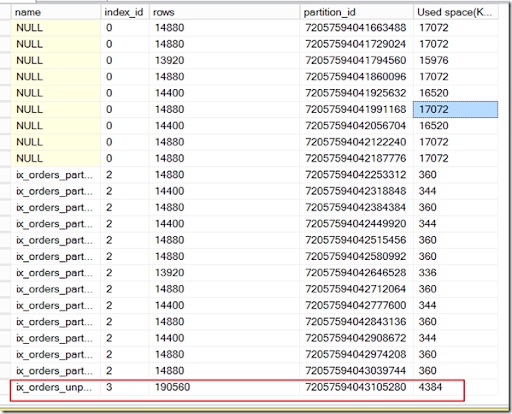## Compare the performance between partition index and non partition index

Case1 with specific query

`Select order_id, orderDate`
`From dbo.orders With (Index(ix_orders_partitioned))`
`Where order_id = 300;`
` `
`Select order_id, orderDate`
`From dbo.orders With (Index(ix_orders_unpartitioned))`
`Where order_id = 300;`

With specific query, the partition index is more expensive than the non partitioned index.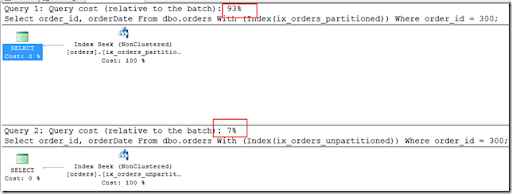Case 2 with Range

`Select order_id, orderDate`
`From dbo.orders With (Index(ix_orders_partitioned))`
`where orderdate between '2011-12-27' and '2012-01-11'`
` `
`Select order_id, orderDate`
`From dbo.orders With (Index(ix_orders_unpartitioned))`
`where orderdate between '2011-12-27' and '2012-01-11'`

Partition index is better than non partition index in this case, because the query is searching for particular date therefore the query optimizer can utilize the partition elimination.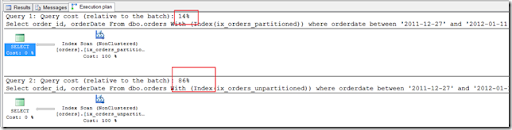## Check the index fragmentation

`SELECT *`
`FROM sys.dm_db_index_physical_stats (DB_ID(),OBJECT_ID(N'dbo.orders'), NULL , NULL, NULL)`
`where object_id=OBJECT_ID(N'dbo.orders')`
`GO`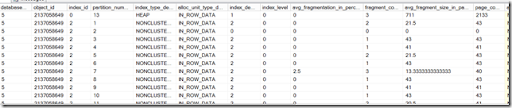## Rebuild the partition index

`ALTER INDEX ix_orders_partitioned`
`ON dbo.orders`
`REBUILD Partition = 5;`

1.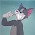2.